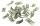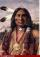Sea water

Mixing 34 kg of sea water with 34 kg rainwater is created water containing 3.4% salt. How many percent sea water contains salt?

Result

x =  6.8 %

Solution:Leave us a comment of example and its solution (i.e. if it is still somewhat unclear...):Be the first to comment!To solve this example are needed these knowledge from mathematics:

Our percentage calculator will help you quickly calculate various typical tasks with percentages. Do you have a linear equation or system of equations and looking for its solution? Or do you have quadratic equation? Tip: Our volume units converter will help you with converion of volume units.

Next similar examples:

1. CakesGrandmother baked cakes. Half of its was poppy, quarter with plum jam and 16 cheesecakes. How many cakes she baked in total?
2. Solutions, mixturesHow many liters of 70% solution we must add to 5 liters of 30% solution to give us a 60% solution?
3. Percents - easyHow many percent is 432 out of 434?
4. Base, percents, valueBase is 344084 which is 100 %. How many percent is 384177?
5. PercentsHow many percents is 900 greater than the number 750?
6. NormThree workers planted 3555 seedlings of tomatoes in one dey. First worked at the standard norm, the second planted 120 seedlings more and the third 135 seedlings more than the first worker. How many seedlings were standard norm?
7. AlleyAlley measured a meters. At the beginning and end are planted poplar. How many we must plant poplars to get the distance between the poplars 15 meters?
8. GoodsTo the market is introducing a new product, the first week is sold at a reduced price. 8 products is sell at an entry price same as 5 products at the normal price. How much % is reduced price less than the current price for this product?
9. New refrigeratorNew refrigerator sells for 1024 USD, Monday will be 25% discount. How much USD will save, and what will be the price?
10. EthnicityShare of ethnicity XY is 26%, which is 1/9 more than in the prewar period. What was the share of that ethnicity in the pre-war period?
11. ExpressionSolve for a specified variable: P=a+4b+3c, for a
12. One-thirdA one-third of unknown number is equal to five times as great as the difference of the same unknown number and number 28. Determine the unknown number.
13. Simple equationSolve for x: 3(x + 2) = x - 18
14. SimplifySimplify the following problem and express as a decimal: 5.68-[5-(2.69+5.65-3.89) /0.5]
15. Equation 29Solve next equation: 2 ( 2x + 3 ) = 8 ( 1 - x) -5 ( x -2 )
16. EquationSolve the equation: 1/2-2/8 = 1/10; Write the result as a decimal number.
17. Equation?